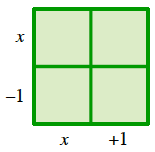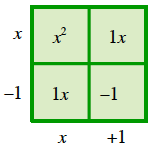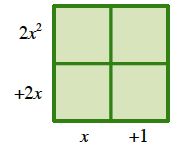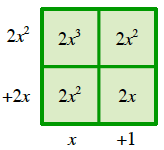### Home > A2C > Chapter 4 > Lesson 4.3.2 > Problem4-155

4-155.

Multiply the expressions in parts (a) through (c) to remove the parentheses.

1. $(x − 1)(x + 1)$

Use a generic rectangle to multiply.

In a rectangle, $\left(\text{length}\right)\left(\text{width}\right) = \text{area}$. Find the area of each small rectangle inside the generic rectangle. 2 by 2 square, left edge labeled, top, x, bottom, minus 1. Bottom edge labeled, left, x, right, + 1.

Added labels to interior: top left, x squared, top right, 1, x, bottom left, 1, x, bottom right, negative 1.

The total area of the generic rectangle, or $x^2−1$2. $2x(x + 1)(x + 1)$

First, multiply $2x$ and $(x+1)$.Then use a generic rectangle to multiply that result by $(x+1)$.

2 by 2 square, left edge labeled, top, 2, x squared, bottom, + 2, x, Bottom edge labeled, left, x, right, + 1.

Added labels to interior: top left, 2, x to the third, top right, 2, x squared, bottom left, 2, x squared, bottom right, 2, x.

$2x^3+4x^2+2x$3. $(x − 1)(x + 1)(x − 2)$

$(x^2−1)(x−2)$
Now use a generic rectangle.

4. Find the $x$- and $y$-intercepts of $y = x^3 − 2x^2 − x + 2$. The factors in part (c) should be useful.

The $x$-intercepts are where $y=0$.

$0=x^3−2x^2−x+2$

The $y$-intercept is where $x=0$:

$y=0^3−2(0)^2−0+2$

$0=(x−1)(x+1)(x−2)$ from part (c).

Using the Zero Product Property: $x−1=0\ \ \text{or}\ \ x+1=0\ \ \text{or}\ \ x−2=0$

$x=1\ \ \text{or}\ \ x=−1\ \ \text{or}\ \ x=2$